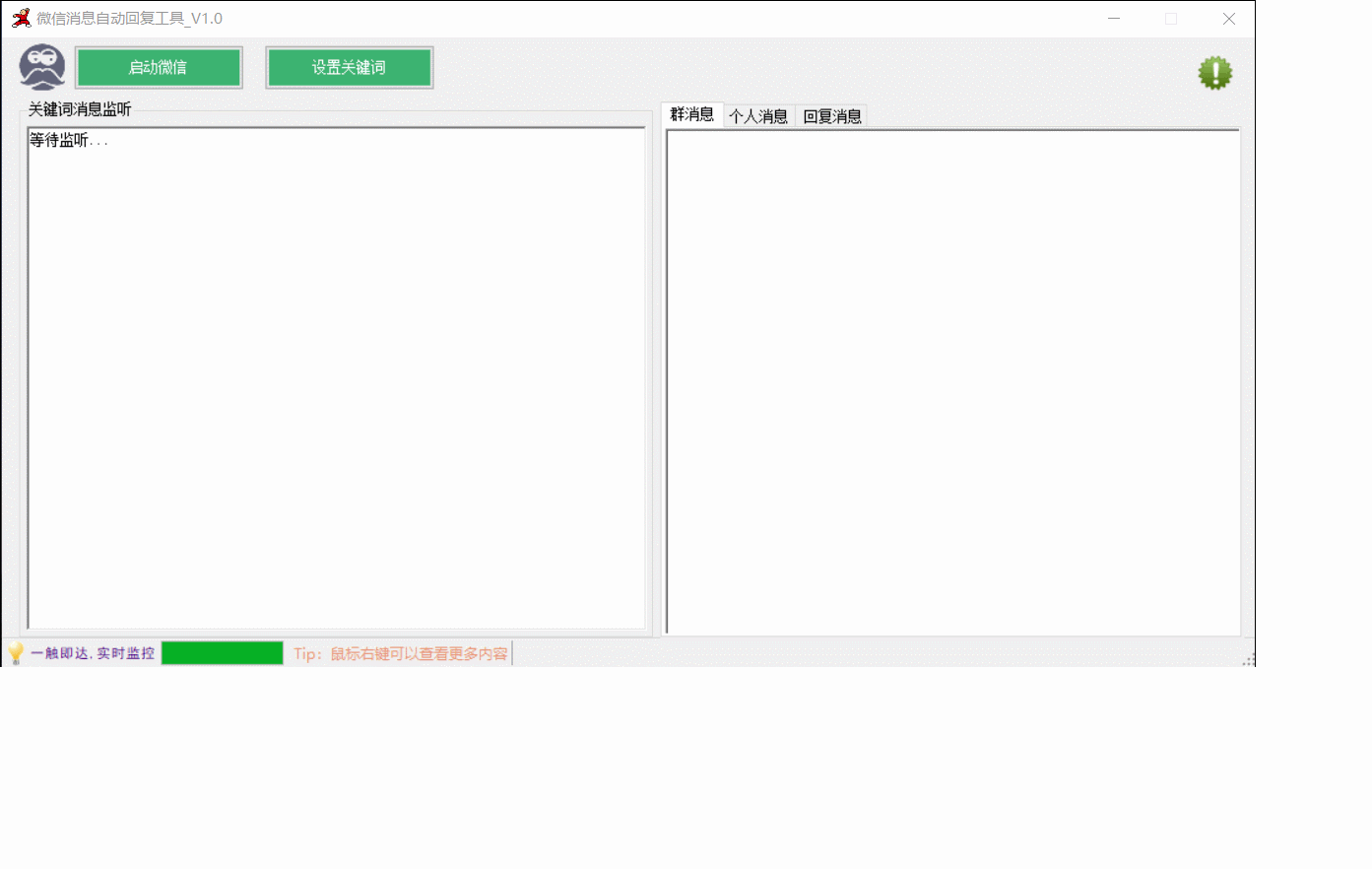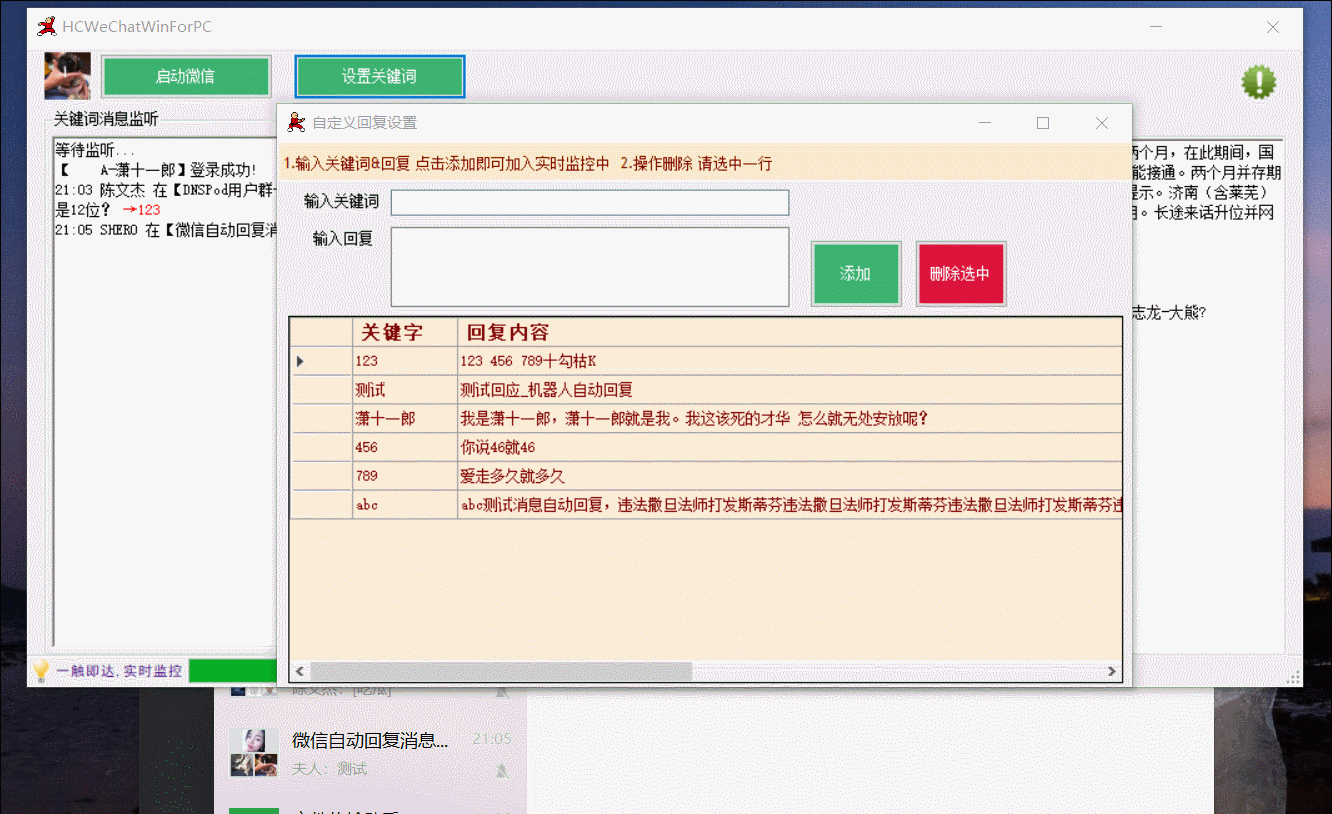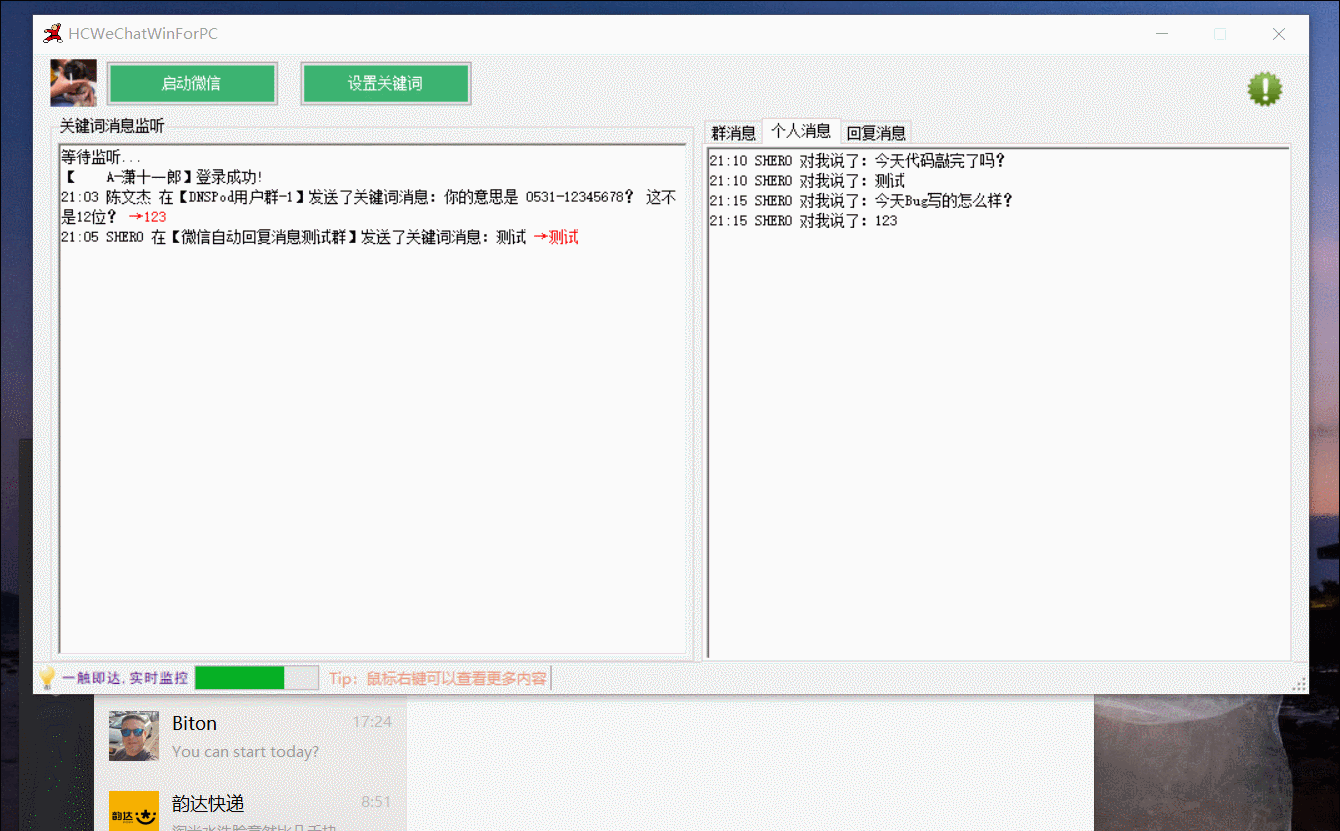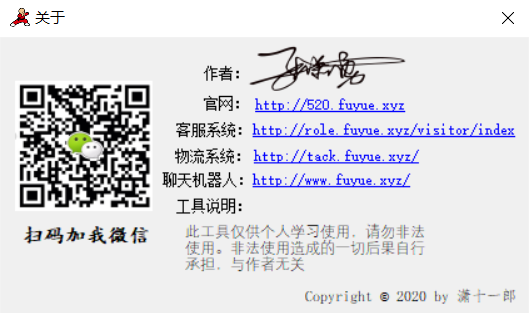# 前言# 步骤分析

1.需要获取到微信登录的"实例"

2.从"实例"中获取出用户信息

3.根据用户信息 获取到当前对话的群/用户 信息

4.根据解析出来的信息 做一些特定事情

用到的一些枚举、类 可以看看

    /// <summary>
///   4 字节布尔值 (<see langword="true" /> != 0, <see langword="false" /> = 0)。
///    这是 Win32 BOOL 类型。
/// </summary>
[__DynamicallyInvokable] Bool = 2,
/// <summary>
///   1 字节有符号整数。
///    可使用此成员将布尔值转换为 1 字节、C 样式的 <see langword="bool" /> (<see langword="true" /> = 1, <see langword="false" /> = 0)。
/// </summary>
[__DynamicallyInvokable] I1 = 3,
/// <summary>1 字节无符号整数。</summary>
[__DynamicallyInvokable] U1 = 4,
/// <summary>2 字节有符号整数。</summary>
[__DynamicallyInvokable] I2 = 5,
/// <summary>2 字节无符号整数。</summary>
[__DynamicallyInvokable] U2 = 6,
/// <summary>4 字节有符号整数。</summary>
[__DynamicallyInvokable] I4 = 7,
/// <summary>4 字节无符号整数。</summary>
[__DynamicallyInvokable] U4 = 8,
/// <summary>8 字节有符号整数。</summary>
[__DynamicallyInvokable] I8 = 9,
/// <summary>8 字节无符号整数。</summary>
[__DynamicallyInvokable] U8 = 10, // 0x0000000A
/// <summary>4 字节浮点数。</summary>
[__DynamicallyInvokable] R4 = 11, // 0x0000000B
/// <summary>8 字节浮点数。</summary>
[__DynamicallyInvokable] R8 = 12, // 0x0000000C
/// <summary>
///   货币类型。
///    在 <see cref="T:System.Decimal" /> 上使用，以将十进制数值作为 COM 货币类型而不是 <see langword="Decimal" /> 封送。
/// </summary>
[__DynamicallyInvokable] Currency = 15, // 0x0000000F
/// <summary>
///   长度前缀为双字节的 Unicode 字符串。
///    可以在 <see cref="T:System.String" /> 数据类型上使用此成员（它是 COM 中的默认字符串）。
/// </summary>
[__DynamicallyInvokable] BStr = 19, // 0x00000013
/// <summary>
///   单字节、以 null 结尾的 ANSI 字符串。
///    可以在 <see cref="T:System.String" /> 和 <see cref="T:System.Text.StringBuilder" /> 数据类型上使用此成员。
/// </summary>
[__DynamicallyInvokable] LPStr = 20, // 0x00000014
/// <summary>一个 2 字节、以 null 结尾的 Unicode 字符串。</summary>
[__DynamicallyInvokable] LPWStr = 21, // 0x00000015
/// <summary>
///   与平台相关的字符串：在 Windows 98 上为 ANSI，在 Windows NT 和 Windows XP 上为 Unicode。
///    该值仅支持平台调用而不支持 COM 互操作，因为不支持导出 <see langword="LPTStr" /> 类型的字符串。
/// </summary>
[__DynamicallyInvokable] LPTStr = 22, // 0x00000016
/// <summary>
///   用于在结构中出现的内联定长字符数组。
///    与 <see cref="F:System.Runtime.InteropServices.UnmanagedType.ByValTStr" /> 一起使用的字符类型由应用于包含结构的 <see cref="T:System.Runtime.InteropServices.StructLayoutAttribute" /> 属性的 <see cref="T:System.Runtime.InteropServices.CharSet" /> 参数确定。
///    应始终使用 <see cref="F:System.Runtime.InteropServices.MarshalAsAttribute.SizeConst" /> 字段来指示数组的大小。
/// </summary>
[__DynamicallyInvokable] ByValTStr = 23, // 0x00000017
/// <summary>
///   COM <see langword="IUnknown" /> 指针。
///    可以在 <see cref="T:System.Object" /> 数据类型上使用此成员。
/// </summary>
[__DynamicallyInvokable] IUnknown = 25, // 0x00000019
/// <summary>
///   COM <see langword="IDispatch" /> 指针（Microsoft Visual Basic 6.0 中的 <see langword="Object" />）。
/// </summary>
[__DynamicallyInvokable] IDispatch = 26, // 0x0000001A
/// <summary>一个用于封送托管格式化类和值类型的 VARIANT。</summary>
[__DynamicallyInvokable] Struct = 27, // 0x0000001B
/// <summary>
///   COM 接口指针。
///    接口的 <see cref="T:System.Guid" /> 可从类元数据获得。
///    如果将此成员应用于类，则可以使用该成员指定确切的接口类型或默认的接口类型。
///    应用于 <see cref="T:System.Object" /> 数据类型时，此成员将产生与 <see cref="F:System.Runtime.InteropServices.UnmanagedType.IUnknown" /> 相同的行为。
/// </summary>
[__DynamicallyInvokable] Interface = 28, // 0x0000001C
/// <summary>
///   <see langword="SafeArray" /> 是自我描述的数组，它带有关联数组数据的类型、秩和界限。
///    可将此成员与 <see cref="F:System.Runtime.InteropServices.MarshalAsAttribute.SafeArraySubType" /> 字段一起使用，以替代默认元素类型。
/// </summary>
[__DynamicallyInvokable] SafeArray = 29, // 0x0000001D
/// <summary>
///   当 <see cref="P:System.Runtime.InteropServices.MarshalAsAttribute.Value" /> 属性被设置为 <see langword="ByValArray" /> 时，必须设置 <see cref="F:System.Runtime.InteropServices.MarshalAsAttribute.SizeConst" /> 字段以指示该数组中的元素数。
///    当需要区分字符串类型时，<see cref="F:System.Runtime.InteropServices.MarshalAsAttribute.ArraySubType" /> 字段可以选择包含数组元素的 <see cref="T:System.Runtime.InteropServices.UnmanagedType" />。
///    此 <see cref="T:System.Runtime.InteropServices.UnmanagedType" /> 只可用于结构中其元素作为字段出现的数组。
/// </summary>
[__DynamicallyInvokable] ByValArray = 30, // 0x0000001E
/// <summary>
///   与平台相关的有符号整数：在 32 位 Windows 上为 4 个字节，在 64 位 Windows 上为 8 个字节。
/// </summary>
[__DynamicallyInvokable] SysInt = 31, // 0x0000001F
/// <summary>
///   与平台相关的无符号整数：在 32 位 Windows 上为 4 个字节，在 64 位 Windows 上为 8 个字节。
/// </summary>
[__DynamicallyInvokable] SysUInt = 32, // 0x00000020
/// <summary>
///   一个值，该值使 Visual Basic 能够更改非托管代码中的字符串，并使结果在托管代码中反映出来。
///    该值仅支持平台调用。
/// </summary>
[__DynamicallyInvokable] VBByRefStr = 34, // 0x00000022
/// <summary>
///   ANSI 字符串是一个带有长度前缀的单字节字符串。
///    可以在 <see cref="T:System.String" /> 数据类型上使用此成员。
/// </summary>
[__DynamicallyInvokable] AnsiBStr = 35, // 0x00000023
/// <summary>
///   一个有长度前缀的与平台相关的 <see langword="char" /> 字符串：在 Windows 98 上为 ANSI，在 Windows NT 上为 Unicode。
///    很少用到这个类似于 BSTR 的成员。
/// </summary>
[__DynamicallyInvokable] TBStr = 36, // 0x00000024
/// <summary>
///   2 字节、OLE 定义的 VARIANT_BOOL 类型 (<see langword="true" /> = -1, <see langword="false" /> = 0)。
/// </summary>
[__DynamicallyInvokable] VariantBool = 37, // 0x00000025
/// <summary>
///   一个可用作 C 样式函数指针的整数。
///    可将此成员用于 <see cref="T:System.Delegate" /> 数据类型或从 <see cref="T:System.Delegate" /> 继承的类型。
/// </summary>
[__DynamicallyInvokable] FunctionPtr = 38, // 0x00000026
/// <summary>
///   一个动态类型，将在运行时确定对象的类型，并将该对象作为所确定的类型进行封送处理。
///    该成员仅对平台调用方法有效。
/// </summary>
[__DynamicallyInvokable] AsAny = 40, // 0x00000028
/// <summary>
///   指向 C 样式数组的第一个元素的指针。
///    当从托管到非托管代码进行封送处理时，该数组的长度由托管数组的长度确定。
///    从非托管到托管代码进行封送处理时，将根据 <see cref="F:System.Runtime.InteropServices.MarshalAsAttribute.SizeConst" /> 和 <see cref="F:System.Runtime.InteropServices.MarshalAsAttribute.SizeParamIndex" /> 字段确定该数组的长度，当需要区分字符串类型时，还可以后跟数组中元素的非托管类型。
/// </summary>
[__DynamicallyInvokable] LPArray = 42, // 0x0000002A
/// <summary>
///   一个指针，它指向用于封送托管格式化类的 C 样式结构。
///    该成员仅对平台调用方法有效。
/// </summary>
[__DynamicallyInvokable] LPStruct = 43, // 0x0000002B
/// <summary>
///   当与 <see cref="F:System.Runtime.InteropServices.MarshalAsAttribute.MarshalType" /> 或 <see cref="F:System.Runtime.InteropServices.MarshalAsAttribute.MarshalTypeRef" /> 字段一起使用时，指定自定义封送拆收器类。
///    可以在任何引用类型上使用此成员。
/// </summary>
[__DynamicallyInvokable] CustomMarshaler = 44, // 0x0000002C
/// <summary>
///   一个本机类型，此类型与 <see cref="F:System.Runtime.InteropServices.UnmanagedType.I4" /> 或 <see cref="F:System.Runtime.InteropServices.UnmanagedType.U4" /> 关联且将导致参数作为导出类型库中的 HRESULT 导出。
/// </summary>
[__DynamicallyInvokable] Error = 45, // 0x0000002D
/// <summary>
///
///     Windows 运行时 接口指针。
///    可以在 <see cref="T:System.Object" /> 数据类型上使用此成员。
/// </summary>
[ComVisible(false), __DynamicallyInvokable] IInspectable = 46, // 0x0000002E
/// <summary>
///
///     Windows 运行时 字符串。
///    可以在 <see cref="T:System.String" /> 数据类型上使用此成员。
/// </summary>
[ComVisible(false), __DynamicallyInvokable] HString = 47, // 0x0000002F
/// <summary>指向 UTF-8 编码字符串的指针。</summary>
[ComVisible(false)] LPUTF8Str = 48, // 0x00000030

   /// <summary>
///   对象的成员顺序依次布局，它们出现导出到非托管内存时的顺序。
///    成员进行布局中指定的封装根据<see cref="F:System.Runtime.InteropServices.StructLayoutAttribute.Pack" />，和可以是不连续。
/// </summary>
[__DynamicallyInvokable] Sequential = 0,
/// <summary>
///   非托管内存中的每个成员的对象的精确位置显式控制，受约束的设置<see cref="F:System.Runtime.InteropServices.StructLayoutAttribute.Pack" />字段。
///    每个成员必须使用<see cref="T:System.Runtime.InteropServices.FieldOffsetAttribute" />指示该字段的类型中的位置。
/// </summary>
[__DynamicallyInvokable] Explicit = 2,
/// <summary>
///   非托管内存中某个对象的成员，则运行时会自动选择适当的布局。
///    无法在托管代码之外公开使用此枚举成员定义的对象。
///    尝试这样做将引发异常。
/// </summary>
[__DynamicallyInvokable] Auto = 3,public static string DownImage(string fileName)
{
string result = "";
try
{
String FileType = "";

byte[] data2 = new byte[data.Length];
int key = 0;
//PNG
for (int i = 0; i <= 0xFF; i++)
{
int dataA = (byte)(data ^ (byte)i);
if ((data ^ (byte)i) == (byte)0x89)
{
if ((data ^ (byte)i) == (byte)0x50)
{
if ((data ^ (byte)i) == (byte)0x4E)
{
if ((data ^ (byte)i) == (byte)0x47)
{
FileType = "png";
key = i;
break;
}
}
}
}
}

if (key == 0)
{
//JPG
for (int i = 0; i <= 0xFF; i++)
{
int dataA = (byte)(data ^ (byte)i);
if ((data ^ (byte)i) == (byte)0xFF)
{
if ((data ^ (byte)i) == (byte)0xD8)
{
if ((data ^ (byte)i) == (byte)0xFF)
{
if ((data ^ (byte)i) == (byte)0xE0 || (data ^ (byte)i) == (byte)0xD9 || (data ^ (byte)i) == (byte)0xE1 || (data ^ (byte)i) == (byte)0xDF)
{
FileType = "jpg";
key = i;
break;
}
}
}
}
}
}

if (key == 0)
{
LogHelper.WriteLog("解密图片失败." + fileName);
if(File.Exists(fileName.Replace("dat", "jpg")))
{
return fileName.Replace("dat", "jpg");
}
if (File.Exists(fileName.Replace("dat", "png")))
{
return fileName.Replace("dat", "png");
}
return "";
}
else
{
//this.textBox2.Text = "0x" + key.ToString("X");
}

for (int i = 0; i < data.Length; i++)
{
data2[i] = (byte)(data[i] ^ key);
}

string picPath = fileName.Replace("dat", "") + FileType;
File.WriteAllBytes(picPath, data2);

ExistPic(picPath);

return picPath;
}
catch (Exception e)
{
LogHelper.WriteLog("解密图片失败!"+ fileName, e);
return "";
}
}
View Code

# 使用说明# 具体实现posted @ 2020-04-25 22:02  潇十一郎  阅读(777)  评论(0编辑  收藏